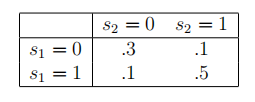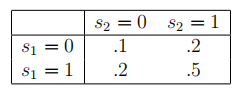CS269I：Incentives in Computer Science 学习笔记 Lecture 17 评分规则和同辈预测（诚实预报和反馈激励）

31 篇文章 0 订阅

Lecture 17 Scoring Rules and Peer Prediction(Incentivizing Honest Forecasts and Feedback)（评分规则和同辈预测（诚实预报和反馈激励））

1 Scoring Rules（评分规则）

1.1 Motivation

1. 你问天气预报：明天下不下雨？
2. 你问民调机构：下次选举是民主党赢还是共和党赢？
3. 你问微软的一个雇员：下一个版本的MS Office会不会延期发售？

1.2 Strictly Proper Scoring Rules（严格正确评分规则）

1. 一个拥有某种“信念”p的预报员，p是在X上的一个概率分布。

2. 一个预报员希望选择她的预报q，使得她在上面的评分函数中得到的分数最大化（qp可能相等也可能不等）。事实上，这并不是很有意义，因为预报员的分数还要取决于真实的结果，这并不由她控制。因此我们假设播报员希望最大化她的期望分数
max ⁡ q E i ∼ p [ S ( q , i ) ] \max _{\mathbf{q}} \mathbf{E}_{i \sim \mathbf{p}}[S(\mathbf{q}, i)]
其中i的期望是由播报员心中对i的分布的“信念”p决定的。

1.3 A Non-Example

S ( q , i ) = q i S(\bold{q},i)=q_i

E [ S c o r e ] = ∑ i ∈ X p i q i E[Score]=\sum_{i\in X} p_iq_i

S ( q , i ) = q i − 1 2 ∑ j ∈ X q j 2 S(\mathbf{q}, i)=q_{i}-\frac{1}{2} \sum_{j \in X} q_{j}^{2}

∑ i ∈ X p i q i − 1 2 ∑ i ∈ X p i ∑ j ∈ X q j 2 \sum_{i \in X} p_{i} q_{i}-\frac{1}{2} \sum_{i \in X} p_{i} \sum_{j \in X} q_{j}^{2}

∂ f ∂ q h = p h − ∑ i ∈ X p i q h \frac{\partial f}{\partial q_{h}}=p_{h}-\sum_{i \in X} p_{i} q_{h}

1.5 The Logarithmic Scoring Rule（对数评分规则）

S ( q , i ) = ln  q i S(\bold{q},i)=\text{ln}\ q_i

E i ∼ p [ S ( q , i ) ] = ∑ i ∈ X p i ln ⁡ q i \mathbf{E}_{i \sim \mathbf{p}}[S(\mathbf{q}, i)]=\sum_{i \in X} p_{i} \ln q_{i}

∂ f ∂ q i = p i q i \frac{\partial f}{\partial q_i}=\frac{p_i}{q_i}

2 Incentivizing Honest Feedback（激励诚实反馈）

2.2 The Model

• 有n个玩家（MOOC里面的n个同学）
• 第i个玩家有一个“信号”si，表示其现在拥有的信息，例如，对作业的质量的真实看法。
• 每个玩家i向机制（例如，mooc平台）提交一个报告ri，ri可以等于或不等于si
• 这个机制向i支付π_i（r1,…,rn）2.3 Output Agreement

Output Agreement：（输出共识）

​ 对于每个玩家i，选择一个随机的玩家j≠i，i的支付πi等于1当且仅当ri=rj，否则等于02.4 The Peer Prediction Mechanism（同伴预测机制）

Peer Prediction（同伴预测）

（a）选取一个随机玩家j≠i

（b）令Dj(ri)表示sj的分布（假设si=ri）

（c）令i的支付πi是S(Dj(ri),rj)

2.5 Implementation Chanllenges（补充挑战）

02-24252

02-25176
02-25102
02-2068
02-1947
02-21274
02-22364
02-22226
02-21133
02-1651
02-18135
02-18241
02-17232
02-06251
02-08152
02-11125
02-10202点击重新获取扫码支付余额充值